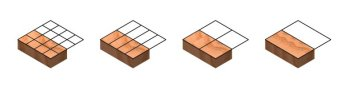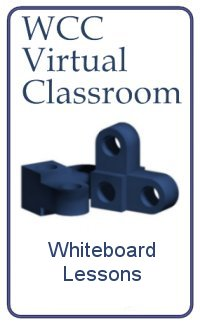Computer-Aided Design Engineering Programming Architectural Design Mechanical Design Civil Design Energy Management Electrical Design

Network
Engineer

Project
Management
 Table of ContentsWhen working with fractions, we need to be able to convert the value to a proportional number so we can add or subtract them. As shown in the image above, by dividing both the numerator and the denominator by two reduces 8/16 to 4/8, then 4/8 to 2/4, and finally 2/4 to 1/2. Therefore, we now know that 8/16 = 4/8 = 2/4 = 1/2. We could have easily gone in the other direction, multiplying both the numerator and the denominator by two never completing the task since both parts of the fraction would go to infinity. At World Class CAD, we use computer applications to solve every type of challenge. Working with fractions is just one. Interested in food density and yield charts, check out these links: Approximate Yields of Fruits and Vegetables from Restaurantcoachingsolutions.com Culinary Math Web Page Title Page Ch.1: Working with Fractions Appendix A: Adding Fractions Appendix B: Subtracting Fractions Appendix C: Multiplying Fractions Appendix D: Dividing Fractions Prime Numbers Exercise Measuring Lab Assignments Tools for Successful Math Training Week 1 Week 2 Week 3 Week 4 Week 5 Week 6 Week 7 Week 8 Reference Multiplication Table Unit Conversion Chart Fraction to Decimal Conversion Chart Using a English Ruler or Metric Scale Quizzes and Tests Week 1 Math Warmup Week 1 Fractions Pretest Week 1 Measuring with Inches and Centimeters Week 1 Measuring Lab Week 1 Measuring Lab Exercise Volume Measurement Quiz 1 Volume Measurement Quiz 2 Week 2 Review - Hospitality Week 2 Test - Hospitality Week 4 Percentage Exercise - Hospitality Week 5 Metric Conversion Review 1 Online Quiz: Metric Conversion Quiz 1 Week 5 Metric Conversion Review 2 Online Quiz: Metric Conversion Quiz 2 Week 5 Metric Conversion Review 3 Online Quiz: Metric Conversion Quiz 3 Week 6 Exercise - Working with Angles Week 6 Scientific Notation Worksheet Week 6 Scientific Notation Activity Week 7 Graphing Lab Week 7 Converting Celsius and Fahrenheit Week 8 Test - Hospitality